# Financial Statement Analysis

### Financial Statement Analysis

Financial statement analysis reviews financial information found on financial statements to make informed decisions about the business. The income statement, statement of retained earnings, balance sheet, and statement of cash flows, among other financial information, can be analyzed. The information obtained from this analysis can benefit decision-making for internal and external stakeholders and can give a company valuable information on overall performance and specific areas for improvement. The analysis can help them with budgeting, deciding where to cut costs, how to increase revenues, and future capital investments opportunities.

When considering the outcomes from analysis, it is important for a company to understand that data produced needs to be compared to others within industry and close competitors. The company should also consider their past experience and how it corresponds to current and future performance expectations. Three common analysis tools are used for decision-making; horizontal analysis, vertical analysis, and financial ratios.

For our discussion of financial statement analysis, we will use Banyan Goods. Banyan Goods is a merchandising company that sells a variety of products. (Figure) shows the comparative income statements and balance sheets for the past two years.

Comparative Income Statements and Balance Sheets.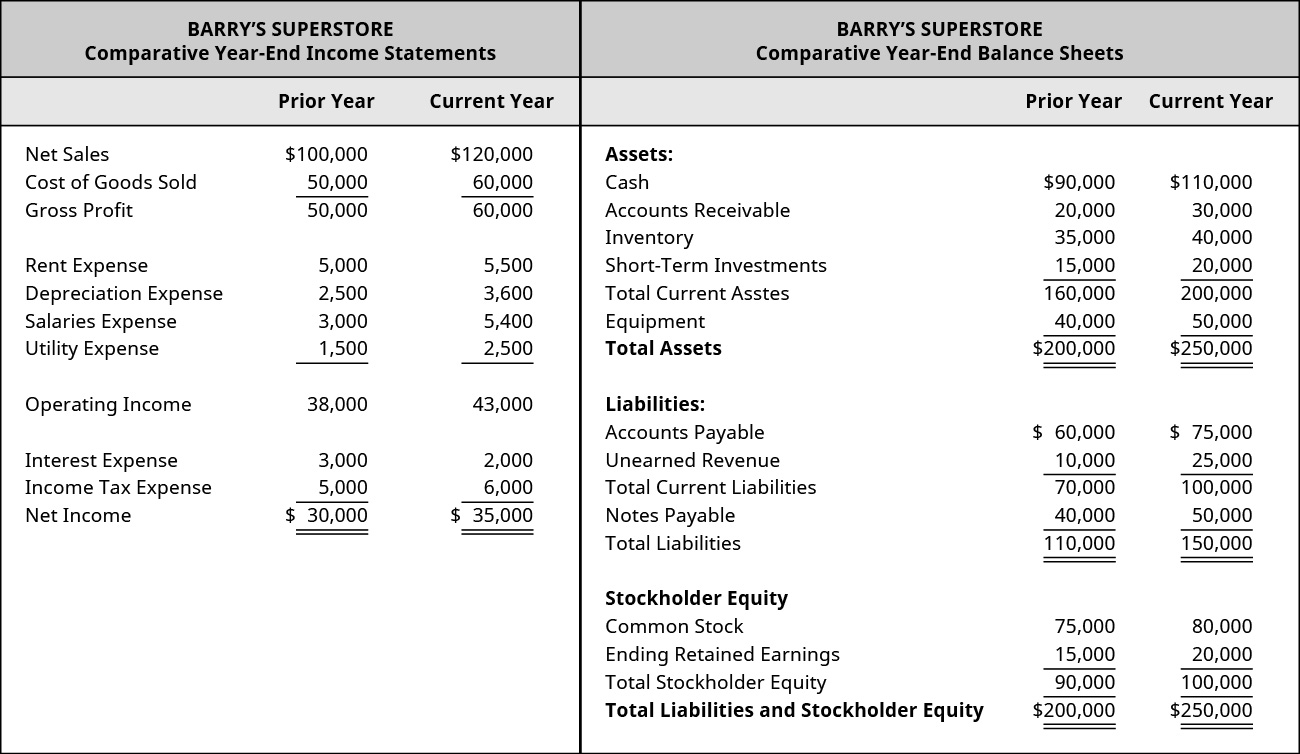Keep in mind that the comparative income statements and balance sheets for Banyan Goods are simplified for our calculations and do not fully represent all the accounts a company could maintain. Let’s begin our analysis discussion by looking at horizontal analysis.

### Horizontal Analysis

Horizontal analysis (also known as trend analysis) looks at trends over time on various financial statement line items. A company will look at one period (usually a year) and compare it to another period. For example, a company may compare sales from their current year to sales from the prior year. The trending of items on these financial statements can give a company valuable information on overall performance and specific areas for improvement. It is most valuable to do horizontal analysis for information over multiple periods to see how change is occurring for each line item. If multiple periods are not used, it can be difficult to identify a trend. The year being used for comparison purposes is called the base year (usually the prior period). The year of comparison for horizontal analysis is analyzed for dollar and percent changes against the base year.

The dollar change is found by taking the dollar amount in the base year and subtracting that from the year of analysis.Using Banyan Goods as our example, if Banyan wanted to compare net sales in the current year (year of analysis) of ?120,000 to the prior year (base year) of ?100,000, the dollar change would be as follows:

$$\text{Dollar change}=?120,000–?1000,000=?20,000$$

The percentage change is found by taking the dollar change, dividing by the base year amount, and then multiplying by 100.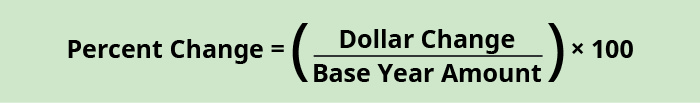Let’s compute the percentage change for Banyan Goods’ net sales.

$$\text{Percentage change}=\left(\frac{?20,000}{?100,000}\right)\phantom{\rule{0.2em}{0ex}}×\phantom{\rule{0.2em}{0ex}}100=20%$$

This means Banyan Goods saw an increase of ?20,000 in net sales in the current year as compared to the prior year, which was a 20% increase. The same dollar change and percentage change calculations would be used for the income statement line items as well as the balance sheet line items. (Figure) shows the complete horizontal analysis of the income statement and balance sheet for Banyan Goods.

Income Statements and Horizontal Analysis.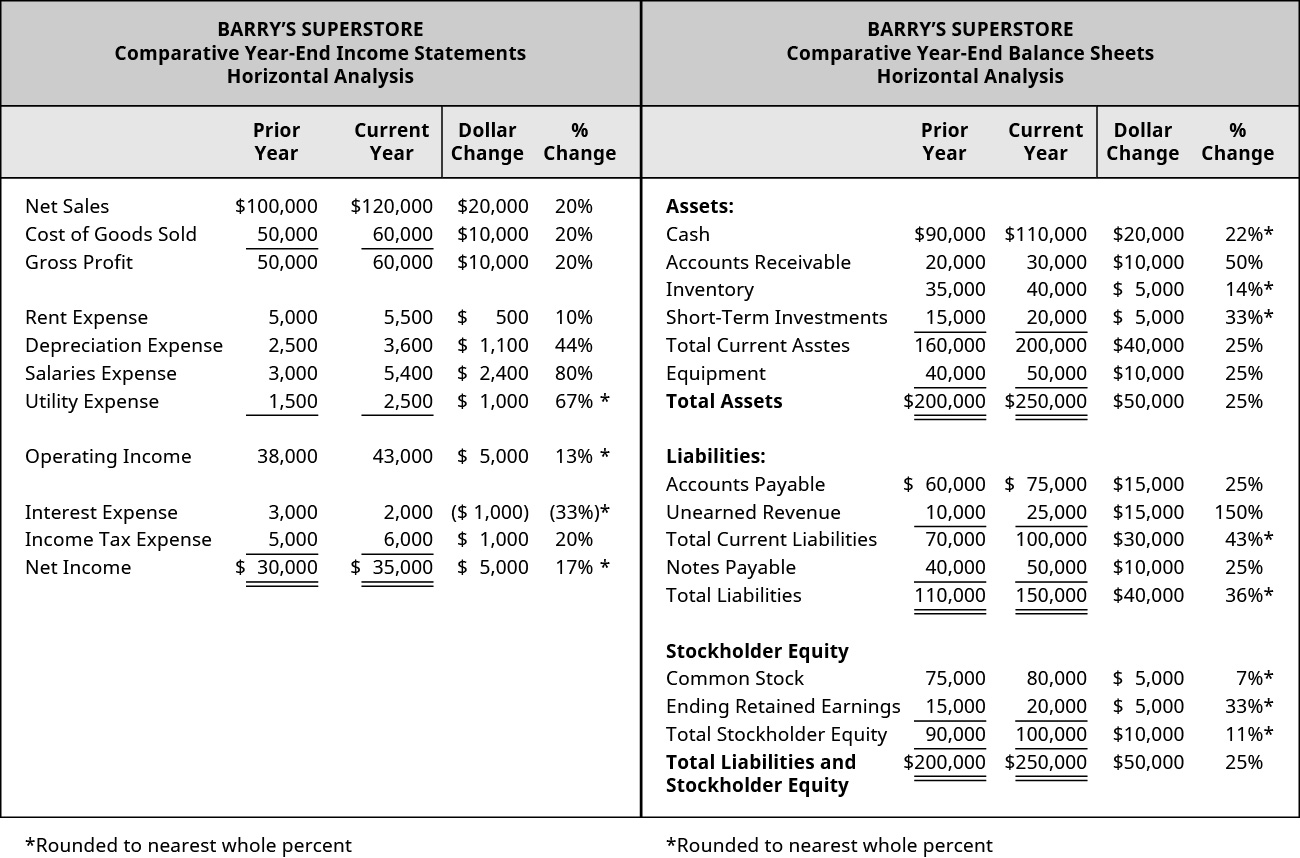Depending on their expectations, Banyan Goods could make decisions to alter operations to produce expected outcomes. For example, Banyan saw a 50% accounts receivable increase from the prior year to the current year. If they were only expecting a 20% increase, they may need to explore this line item further to determine what caused this difference and how to correct it going forward. It could possibly be that they are extending credit more readily than anticipated or not collecting as rapidly on outstanding accounts receivable. The company will need to further examine this difference before deciding on a course of action. Another method of analysis Banyan might consider before making a decision is vertical analysis.

### Vertical Analysis

Vertical analysis shows a comparison of a line item within a statement to another line item within that same statement. For example, a company may compare cash to total assets in the current year. This allows a company to see what percentage of cash (the comparison line item) makes up total assets (the other line item) during the period. This is different from horizontal analysis, which compares across years. Vertical analysis compares line items within a statement in the current year. This can help a business to know how much of one item is contributing to overall operations. For example, a company may want to know how much inventory contributes to total assets. They can then use this information to make business decisions such as preparing the budget, cutting costs, increasing revenues, or capital investments.

The company will need to determine which line item they are comparing all items to within that statement and then calculate the percentage makeup. These percentages are considered common-size because they make businesses within industry comparable by taking out fluctuations for size. It is typical for an income statement to use net sales (or sales) as the comparison line item. This means net sales will be set at 100% and all other line items within the income statement will represent a percentage of net sales.

On the balance sheet, a company will typically look at two areas: (1) total assets, and (2) total liabilities and stockholders’ equity. Total assets will be set at 100% and all assets will represent a percentage of total assets. Total liabilities and stockholders’ equity will also be set at 100% and all line items within liabilities and equity will be represented as a percentage of total liabilities and stockholders’ equity. The line item set at 100% is considered the base amount and the comparison line item is considered the comparison amount. The formula to determine the common-size percentage is: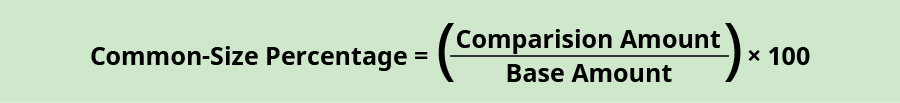For example, if Banyan Goods set total assets as the base amount and wanted to see what percentage of total assets were made up of cash in the current year, the following calculation would occur.

$$\text{Common-size percentage}=\left(\frac{?110,000}{?250,000}\right)\phantom{\rule{0.2em}{0ex}}×\phantom{\rule{0.2em}{0ex}}100=44%$$

Cash in the current year is ?110,000 and total assets equal ?250,000, giving a common-size percentage of 44%. If the company had an expected cash balance of 40% of total assets, they would be exceeding expectations. This may not be enough of a difference to make a change, but if they notice this deviates from industry standards, they may need to make adjustments, such as reducing the amount of cash on hand to reinvest in the business. (Figure) shows the common-size calculations on the comparative income statements and comparative balance sheets for Banyan Goods.

Income Statements and Vertical Analysis.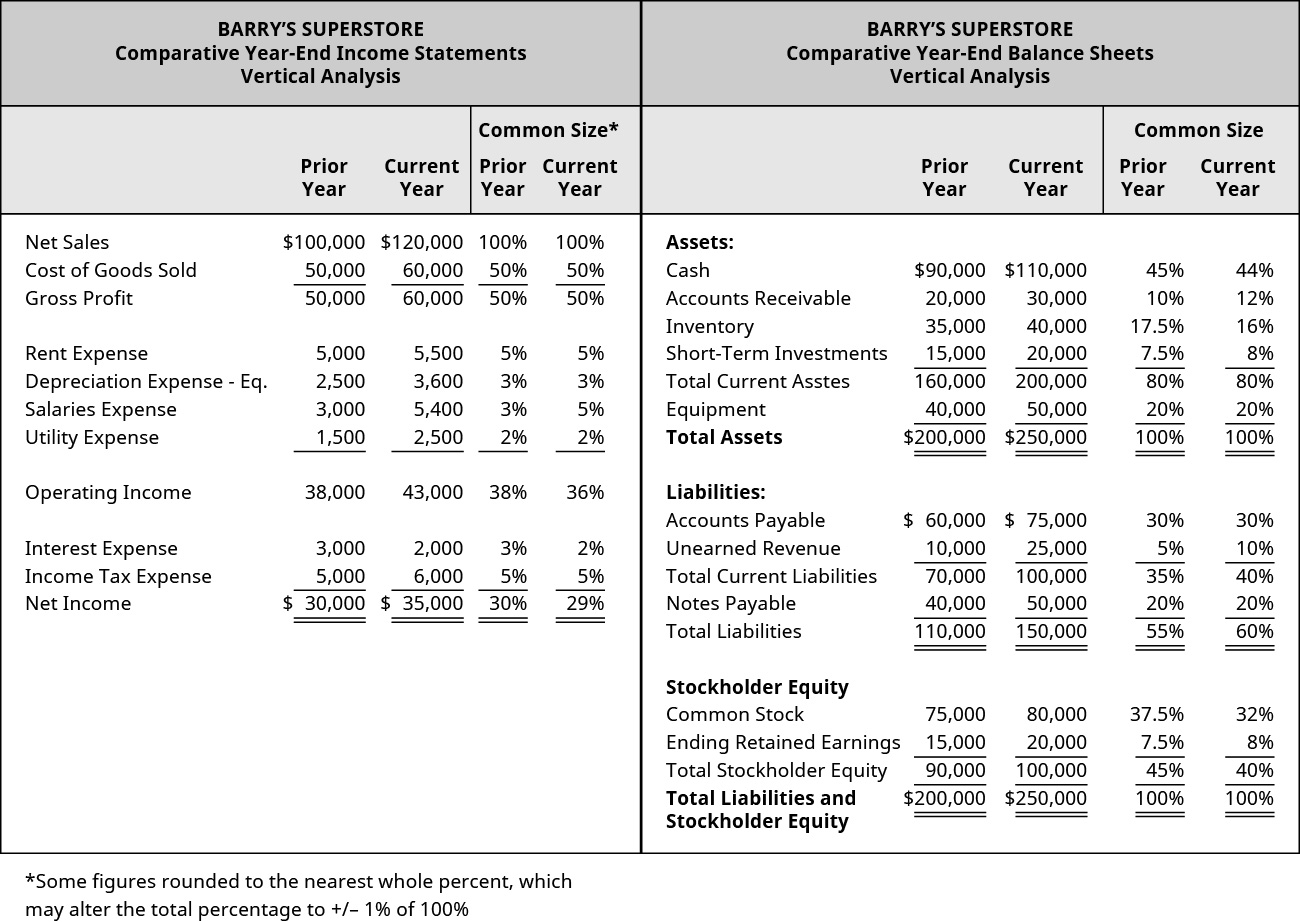Even though vertical analysis is a statement comparison within the same year, Banyan can use information from the prior year’s vertical analysis to make sure the business is operating as expected. For example, unearned revenues increased from the prior year to the current year and made up a larger portion of total liabilities and stockholders’ equity. This could be due to many factors, and Banyan Goods will need to examine this further to see why this change has occurred. Let’s turn to financial statement analysis using financial ratios.

### Overview of Financial Ratios

Financial ratios help both internal and external users of information make informed decisions about a company. A stakeholder could be looking to invest, become a supplier, make a loan, or alter internal operations, among other things, based in part on the outcomes of ratio analysis. The information resulting from ratio analysis can be used to examine trends in performance, establish benchmarks for success, set budget expectations, and compare industry competitors. There are four main categories of ratios: liquidity, solvency, efficiency, and profitability. Note that while there are more ideal outcomes for some ratios, the industry in which the business operates can change the influence each of these outcomes has over stakeholder decisions. (You will learn more about ratios, industry standards, and ratio interpretation in advanced accounting courses.)

#### Liquidity Ratios

Liquidity ratios show the ability of the company to pay short-term obligations if they came due immediately with assets that can be quickly converted to cash. This is done by comparing current assets to current liabilities. Lenders, for example, may consider the outcomes of liquidity ratios when deciding whether to extend a loan to a company. A company would like to be liquid enough to manage any currently due obligations but not too liquid where they may not be effectively investing in growth opportunities. Three common liquidity measurements are working capital, current ratio, and quick ratio.

##### Working Capital

Working capital measures the financial health of an organization in the short-term by finding the difference between current assets and current liabilities. A company will need enough current assets to cover current liabilities; otherwise, they may not be able to continue operations in the future. Before a lender extends credit, they will review the working capital of the company to see if the company can meet their obligations. A larger difference signals that a company can cover their short-term debts and a lender may be more willing to extend the loan. On the other hand, too large of a difference may indicate that the company may not be correctly using their assets to grow the business. The formula for working capital is:Using Banyan Goods, working capital is computed as follows for the current year:

$$\text{Working capital}=?200,000–?100,000=?100,000$$

In this case, current assets were ?200,000, and current liabilities were ?100,000. Current assets were far greater than current liabilities for Banyan Goods and they would easily be able to cover short-term debt.

The dollar value of the difference for working capital is limited given company size and scope. It is most useful to convert this information to a ratio to determine the company’s current financial health. This ratio is the current ratio.

##### Current Ratio

Working capital expressed as a ratio is the current ratio. The current ratio considers the amount of current assets available to cover current liabilities. The higher the current ratio, the more likely the company can cover its short-term debt. The formula for current ratio is:The current ratio in the current year for Banyan Goods is:

$$\text{Current ratio}=\left(\frac{?200,000}{?100,000}\right)=\text{2 or 2:1}$$

A 2:1 ratio means the company has twice as many current assets as current liabilities; typically, this would be plenty to cover obligations. This may be an acceptable ratio for Banyan Goods, but if it is too high, they may want to consider using those assets in a different way to grow the company.

##### Quick Ratio

The quick ratio, also known as the acid-test ratio, is similar to the current ratio except current assets are more narrowly defined as the most liquid assets, which exclude inventory and prepaid expenses. The conversion of inventory and prepaid expenses to cash can sometimes take more time than the liquidation of other current assets. A company will want to know what they have on hand and can use quickly if an immediate obligation is due. The formula for the quick ratio is:The quick ratio for Banyan Goods in the current year is:

$$\text{Quick ratio}=\left(\frac{?110,000+?20,000+?30,000}{?100,000}\right)=\text{1.6 or 1.6:1}$$

A 1.6:1 ratio means the company has enough quick assets to cover current liabilities.

Another category of financial measurement uses solvency ratios.

#### Solvency Ratios

Solvency implies that a company can meet its long-term obligations and will likely stay in business in the future. To stay in business the company must generate more revenue than debt in the long-term. Meeting long-term obligations includes the ability to pay any interest incurred on long-term debt. Two main solvency ratios are the debt-to-equity ratio and the times interest earned ratio.

##### Debt to Equity Ratio

The debt-to-equity ratio shows the relationship between debt and equity as it relates to business financing. A company can take out loans, issue stock, and retain earnings to be used in future periods to keep operations running. It is less risky and less costly to use equity sources for financing as compared to debt resources. This is mainly due to interest expense repayment that a loan carries as opposed to equity, which does not have this requirement. Therefore, a company wants to know how much debt and equity contribute to its financing. Ideally, a company would prefer more equity than debt financing. The formula for the debt to equity ratio is:The information needed to compute the debt-to-equity ratio for Banyan Goods in the current year can be found on the balance sheet.

$$\text{Debt-to-equity ratio}=\left(\frac{?150,000}{?100,000}\right)=\text{1.5 or 1.5:1}$$

This means that for every ?1 of equity contributed toward financing, ?1.50 is contributed from lenders. This would be a concern for Banyan Goods. This could be a red flag for potential investors that the company could be trending toward insolvency. Banyan Goods might want to get the ratio below 1:1 to improve their long-term business viability.

##### Times Interest Earned Ratio

Time interest earned measures the company’s ability to pay interest expense on long-term debt incurred. This ability to pay is determined by the available earnings before interest and taxes (EBIT) are deducted. These earnings are considered the operating income. Lenders will pay attention to this ratio before extending credit. The more times over a company can cover interest, the more likely a lender will extend long-term credit. The formula for times interest earned is:The information needed to compute times interest earned for Banyan Goods in the current year can be found on the income statement.

$$\text{Times interest earned}=\left(\frac{?43,000}{?2,000}\right)=21.5\phantom{\rule{0.2em}{0ex}}\text{times}$$

The ?43,000 is the operating income, representing earnings before interest and taxes. The 21.5 times outcome suggests that Banyan Goods can easily repay interest on an outstanding loan and creditors would have little risk that Banyan Goods would be unable to pay.

Another category of financial measurement uses efficiency ratios.

#### Efficiency Ratios

Efficiency shows how well a company uses and manages their assets. Areas of importance with efficiency are management of sales, accounts receivable, and inventory. A company that is efficient typically will be able to generate revenues quickly using the assets it acquires. Let’s examine four efficiency ratios: accounts receivable turnover, total asset turnover, inventory turnover, and days’ sales in inventory.

##### Accounts Receivable Turnover

Accounts receivable turnover measures how many times in a period (usually a year) a company will collect cash from accounts receivable. A higher number of times could mean cash is collected more quickly and that credit customers are of high quality. A higher number is usually preferable because the cash collected can be reinvested in the business at a quicker rate. A lower number of times could mean cash is collected slowly on these accounts and customers may not be properly qualified to accept the debt. The formula for accounts receivable turnover is: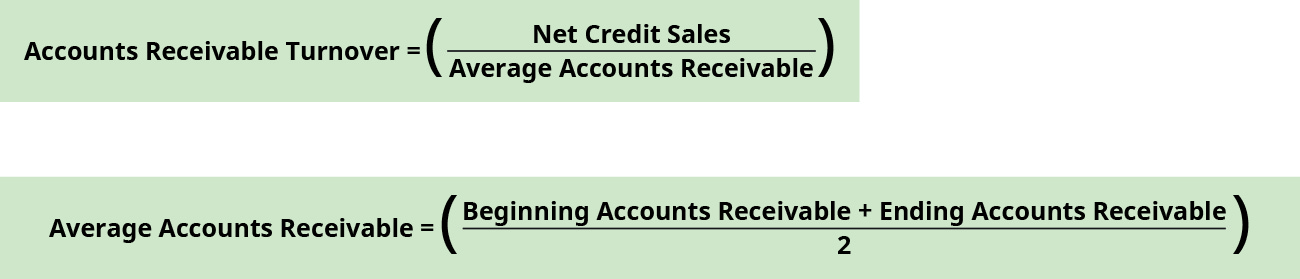Many companies do not split credit and cash sales, in which case net sales would be used to compute accounts receivable turnover. Average accounts receivable is found by dividing the sum of beginning and ending accounts receivable balances found on the balance sheet. The beginning accounts receivable balance in the current year is taken from the ending accounts receivable balance in the prior year.

When computing the accounts receivable turnover for Banyan Goods, let’s assume net credit sales make up ?100,000 of the ?120,000 of the net sales found on the income statement in the current year.

$$\begin{array}{ccc}\hfill \text{Average accounts receivable}& =\hfill & \frac{?20,000+?30,000}{2}=?25,000\hfill \\ \hfill \text{Accounts receivable turnover}& =\hfill & \frac{?100,000}{?25,000}=\text{4 times}\hfill \end{array}$$

An accounts receivable turnover of four times per year may be low for Banyan Goods. Given this outcome, they may want to consider stricter credit lending practices to make sure credit customers are of a higher quality. They may also need to be more aggressive with collecting any outstanding accounts.

##### Total Asset Turnover

Total asset turnover measures the ability of a company to use their assets to generate revenues. A company would like to use as few assets as possible to generate the most net sales. Therefore, a higher total asset turnover means the company is using their assets very efficiently to produce net sales. The formula for total asset turnover is: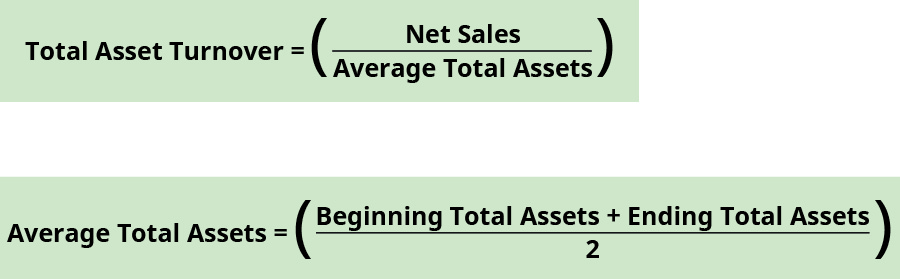Average total assets are found by dividing the sum of beginning and ending total assets balances found on the balance sheet. The beginning total assets balance in the current year is taken from the ending total assets balance in the prior year.

Banyan Goods’ total asset turnover is:

$$\begin{array}{ccc}\hfill \text{Average total assets}& =\hfill & \frac{?200,000+?250,000}{2}=?225,000\hfill \\ \hfill \text{Total assets turnover}& =\hfill & \frac{?120,000}{?225,000}=0.53\phantom{\rule{0.2em}{0ex}}\text{times (rounded)}\hfill \end{array}$$

The outcome of 0.53 means that for every ?1 of assets, ?0.53 of net sales are generated. Over time, Banyan Goods would like to see this turnover ratio increase.

##### Inventory Turnover

Inventory turnover measures how many times during the year a company has sold and replaced inventory. This can tell a company how well inventory is managed. A higher ratio is preferable; however, an extremely high turnover may mean that the company does not have enough inventory available to meet demand. A low turnover may mean the company has too much supply of inventory on hand. The formula for inventory turnover is: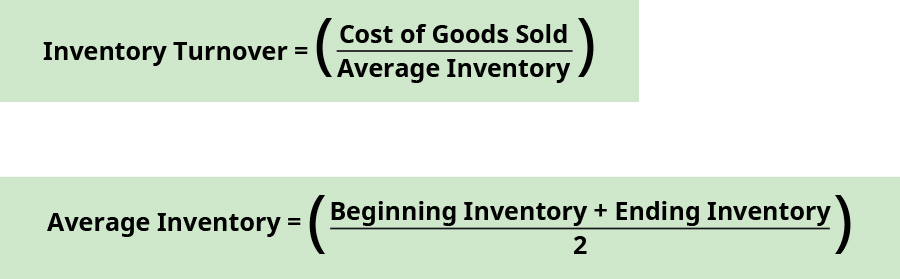Cost of goods sold for the current year is found on the income statement. Average inventory is found by dividing the sum of beginning and ending inventory balances found on the balance sheet. The beginning inventory balance in the current year is taken from the ending inventory balance in the prior year.

Banyan Goods’ inventory turnover is:

$$\begin{array}{ccc}\hfill \text{Average inventory}& =\hfill & \frac{?35,000+?40,000}{2}=?37,500\hfill \\ \hfill \text{Inventory turnover}& =\hfill & \frac{?60,000}{?37,500}=1.6\phantom{\rule{0.2em}{0ex}}\text{times}\hfill \end{array}$$

1.6 times is a very low turnover rate for Banyan Goods. This may mean the company is maintaining too high an inventory supply to meet a low demand from customers. They may want to decrease their on-hand inventory to free up more liquid assets to use in other ways.

##### Days’ Sales in Inventory

Days’ sales in inventory expresses the number of days it takes a company to turn inventory into sales. This assumes that no new purchase of inventory occurred within that time period. The fewer the number of days, the more quickly the company can sell its inventory. The higher the number of days, the longer it takes to sell its inventory. The formula for days’ sales in inventory is:Banyan Goods’ days’ sales in inventory is:

$$\text{Days’ sales in inventory}=\left(\frac{?40,000}{?60,000}\right)\phantom{\rule{0.2em}{0ex}}×\phantom{\rule{0.2em}{0ex}}365=243\phantom{\rule{0.2em}{0ex}}\text{days (rounded)}$$

243 days is a long time to sell inventory. While industry dictates what is an acceptable number of days to sell inventory, 243 days is unsustainable long-term. Banyan Goods will need to better manage their inventory and sales strategies to move inventory more quickly.

The last category of financial measurement examines profitability ratios.

#### Profitability Ratios

Profitability considers how well a company produces returns given their operational performance. The company needs to leverage its operations to increase profit. To assist with profit goal attainment, company revenues need to outweigh expenses. Let’s consider three profitability measurements and ratios: profit margin, return on total assets, and return on equity.

##### Profit Margin

Profit margin represents how much of sales revenue has translated into income. This ratio shows how much of each ?1 of sales is returned as profit. The larger the ratio figure (the closer it gets to 1), the more of each sales dollar is returned as profit. The portion of the sales dollar not returned as profit goes toward expenses. The formula for profit margin is:For Banyan Goods, the profit margin in the current year is:

$$\text{Profit margin}=\left(\frac{?35,000}{?120,000}\right)=0.29\phantom{\rule{0.2em}{0ex}}\text{(rounded)}\phantom{\rule{0.2em}{0ex}}\text{or}\phantom{\rule{0.2em}{0ex}}29%$$

This means that for every dollar of sales, ?0.29 returns as profit. If Banyan Goods thinks this is too low, the company would try and find ways to reduce expenses and increase sales.

##### Return on Total Assets

The return on total assets measures the company’s ability to use its assets successfully to generate a profit. The higher the return (ratio outcome), the more profit is created from asset use. Average total assets are found by dividing the sum of beginning and ending total assets balances found on the balance sheet. The beginning total assets balance in the current year is taken from the ending total assets balance in the prior year. The formula for return on total assets is: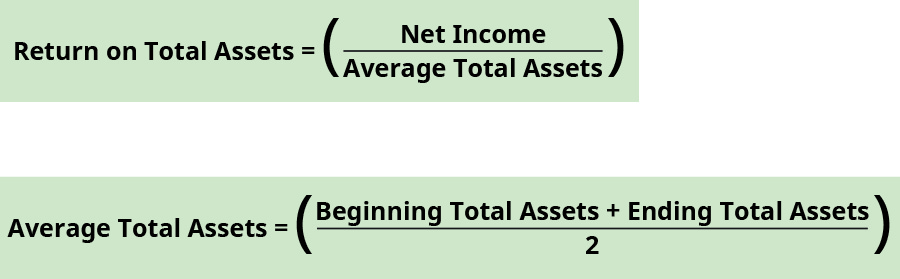For Banyan Goods, the return on total assets for the current year is:

$$\begin{array}{ccc}\hfill \text{Average total assets}& =\hfill & \frac{?200,000+?250,000}{2}=?225,000\hfill \\ \hfill \text{Return on total assets}& =\hfill & \frac{?35,000}{?225,000}=0.16\phantom{\rule{0.2em}{0ex}}\text{(rounded) or 16%}\hfill \end{array}$$

The higher the figure, the better the company is using its assets to create a profit. Industry standards can dictate what is an acceptable return.

##### Return on Equity

Return on equity measures the company’s ability to use its invested capital to generate income. The invested capital comes from stockholders investments in the company’s stock and its retained earnings and is leveraged to create profit. The higher the return, the better the company is doing at using its investments to yield a profit. The formula for return on equity is: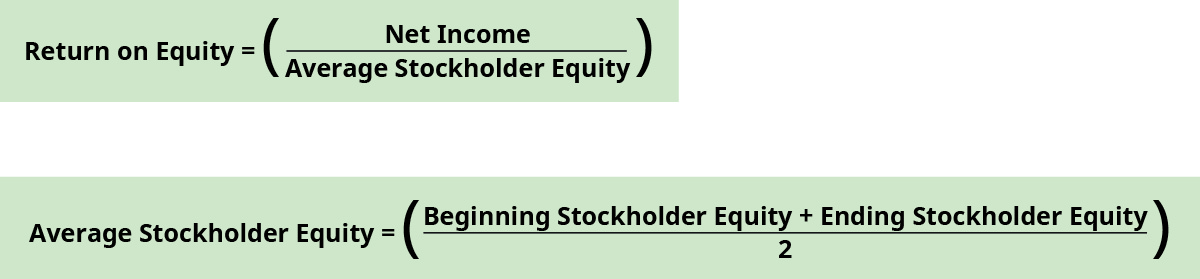Average stockholders’ equity is found by dividing the sum of beginning and ending stockholders’ equity balances found on the balance sheet. The beginning stockholders’ equity balance in the current year is taken from the ending stockholders’ equity balance in the prior year. Keep in mind that the net income is calculated after preferred dividends have been paid.

For Banyan Goods, we will use the net income figure and assume no preferred dividends have been paid. The return on equity for the current year is:

$$\begin{array}{ccc}\hfill \text{Average stockholder equity}& =\hfill & \frac{?90,000+?100,000}{2}=?95,000\hfill \\ \hfill \text{Return on equity}& =\hfill & \frac{?35,000}{?95,000}=0.37\phantom{\rule{0.2em}{0ex}}\text{(rounded) or 37%}\hfill \end{array}$$

The higher the figure, the better the company is using its investments to create a profit. Industry standards can dictate what is an acceptable return.

There are several advantages and disadvantages to financial statement analysis. Financial statement analysis can show trends over time, which can be helpful in making future business decisions. Converting information to percentages or ratios eliminates some of the disparity between competitor sizes and operating abilities, making it easier for stakeholders to make informed decisions. It can assist with understanding the makeup of current operations within the business, and which shifts need to occur internally to increase productivity.

A stakeholder needs to keep in mind that past performance does not always dictate future performance. Attention must be given to possible economic influences that could skew the numbers being analyzed, such as inflation or a recession. Additionally, the way a company reports information within accounts may change over time. For example, where and when certain transactions are recorded may shift, which may not be readily evident in the financial statements.

A company that wants to budget properly, control costs, increase revenues, and make long-term expenditure decisions may want to use financial statement analysis to guide future operations. As long as the company understands the limitations of the information provided, financial statement analysis is a good tool to predict growth and company financial strength.